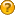###Author Topic: Calculate the Sharpe Ratio of one pair while multiple ones are in the portfolio?  (Read 93 times)

#### JackBeach

• Newbie
•• Posts: 23
• Karma: +1/-0##### Calculate the Sharpe Ratio of one pair while multiple ones are in the portfolio?
« on: February 05, 2023, 05:33:00 am »
Hello all,

Is it possible to calculate the Sharpe Ratio of one pair while multiple ones are present and active in the portfolio?

Say (For the sake of example) I'm having 2 pairs in my portfolio: F/GM and PEP/KO.
I want to calculate the Sharpe Ratio of PEP/KO only.

Is that possible to do so, since both pairs are somewhat linked to my portfolio result?
I don't see a way to do what I want, except having only 1 pair in my portfolio so that it's possible to calculate the Sharpe Ratio since I will know exactly the performance of that said pair because it's going to be straightforward by using the Net Liquidity value, which of course, is suicidal.

By the way, the idea is to calculate the Sharpe Ratio using the paper/live trading account, not using the back tester.